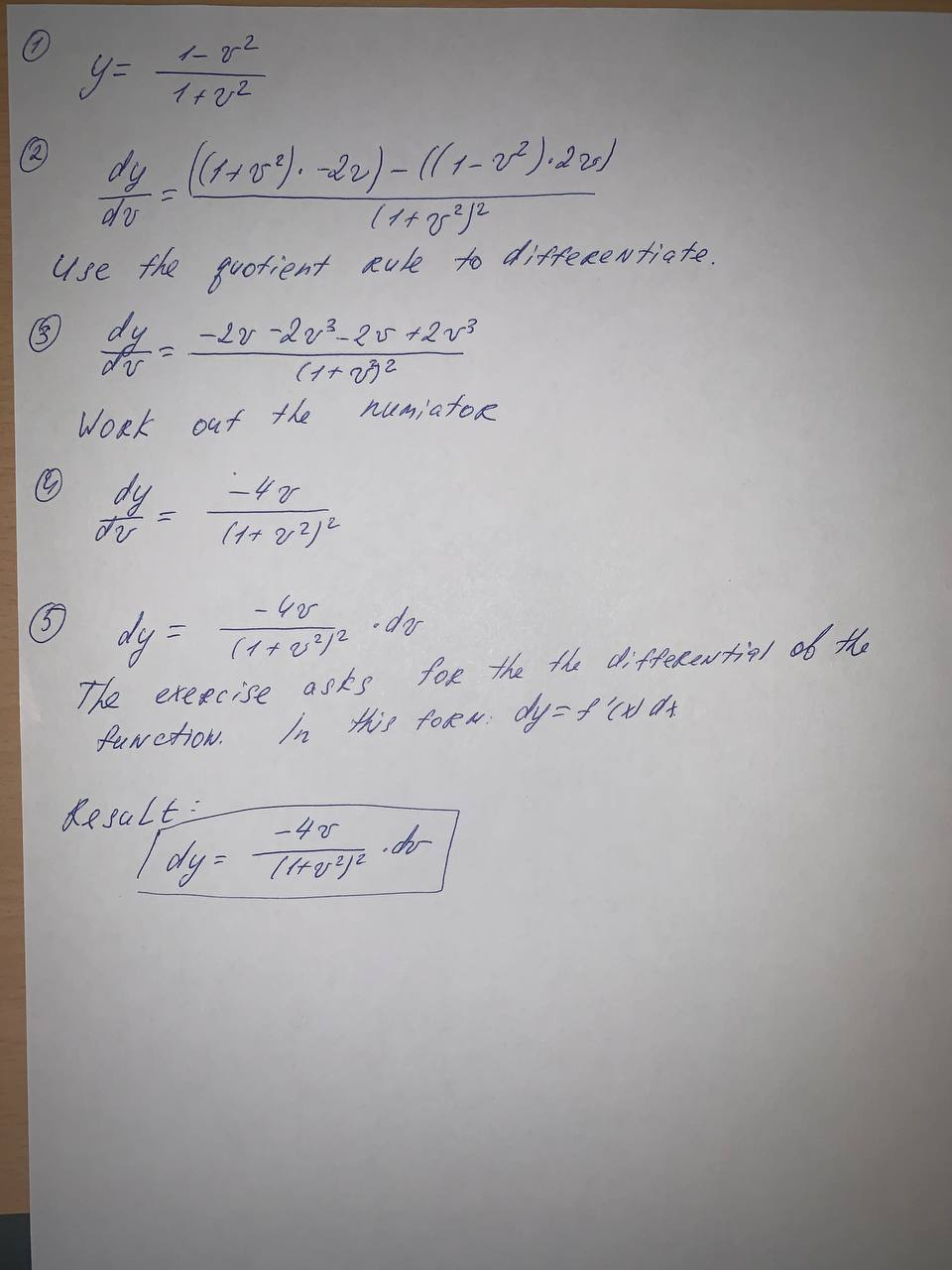# Find the differential of each function.(a) y = \tan \sqrt{t}(b) y= \frac{1-v^2}Kaycee Roche 2021-09-06 Answered

Find the differential of each function.
(a) $$y = \tan \sqrt{t}$$
(b) $$y= \frac{1-v^2}{1+v^2}$$

• Questions are typically answered in as fast as 30 minutes

### Plainmath recommends

• Get a detailed answer even on the hardest topics.
• Ask an expert for a step-by-step guidance to learn to do it yourself.faldduE